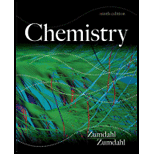# For the following half-reaction, = −2.07 V: A1F 6 3 − ( a q ) + 3e − → Al ( s ) + 6F − ( a q ) Using data from Table 17-1, calculate the equilibrium constant at 25°C for the reaction A1 3+ ( a q ) + 6F − ( a q ) ⇌ A1F 6 3 − ( a q ) K = ?### Chemistry

9th Edition
Steven S. Zumdahl
Publisher: Cengage Learning
ISBN: 9781133611097### Chemistry

9th Edition
Steven S. Zumdahl
Publisher: Cengage Learning
ISBN: 9781133611097

#### Solutions

Chapter
Section
Chapter 18, Problem 90E
Textbook Problem

## Expert Solution

### Want to see the full answer?

Check out a sample textbook solution.See solution

### Want to see this answer and more?

Experts are waiting 24/7 to provide step-by-step solutions in as fast as 30 minutes!*

See Solution

*Response times vary by subject and question complexity. Median response time is 34 minutes and may be longer for new subjects.